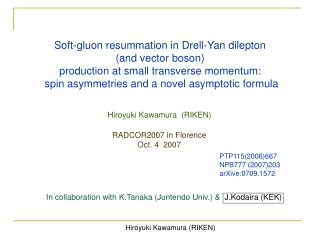DownloadDownload PresentationSoft-gluon resummation in Drell-Yan dilepton (and vector boson)

# Soft-gluon resummation in Drell-Yan dilepton (and vector boson)

Download Presentation## Soft-gluon resummation in Drell-Yan dilepton (and vector boson)

- - - - - - - - - - - - - - - - - - - - - - - - - - - E N D - - - - - - - - - - - - - - - - - - - - - - - - - - -
##### Presentation Transcript

1. Soft-gluon resummation in Drell-Yan dilepton (and vector boson) production at small transverse momentum: spin asymmetries and a novel asymptotic formula Hiroyuki Kawamura (RIKEN) RADCOR2007 in Florence Oct. 4 2007 PTP115(2006)667 NPB777 (2007)203 arXive:0709.1572 In collaboration with K.Tanaka (Juntendo Univ.) & J.Kodaira (KEK)

2. Jiro Kodaira (1951-2006) RADCOR2005 in Shonan (Oct. 2005)

3. Transversely polarized Drell-Yan process • tDY (RHIC, J-PARC, GSI,…) ♠ double spin asymmetry: • Transversity — twist-2, chiral-odd distribution function • No gluon contribution (→ NS-type evolution) • Not measured in inclusive DIS Future DY data can provide an direct access to δq.

4. Double spin asymmetry at small QT • ATT for the “QT-integrated” cross sections at NLO RHIC (PP) : Martin, Shäfer, Stratmann,Vogelsang (’99) GSI (PP-bar ) : Shimizu, Sterman, Yokoya,Vogelsang (’05) (threshold resummation) Barone et al. (’06) • ATT(QT) at small QT • A bulk of dileptons is produced. • Soft gluon corrections are dominant :“universal” • →Extraction of δq(x) can be simpler. — resummation of “recoil logs” — spin asymmetry with soft gluon resummation

5. QT distributions at LO Kodaira, Shimizu, Tanaka, HK (’06) • Drell-Yan process with transverse polarization φ: azimuthal angle of one of the leptons → phase space integral with φ dependence (difficult in D-dimension) ♠ soft/col. singularity appear only at QT=0. ex. D-dim. 4-dim. • QT distribution at LO Altarelli, Ellis,Greco,Martinelli (’84)

6. NLL resummation for tDY Kodaira, Shimizu, Tanaka, HK (’06) Resummed part: double Mellin space pdf b : impact parameter • coeff. function • Sudakov factor LL NLL universal NLL • evolution op. Grazzini, de Florian (‘00)

7. NLL resummation for tDY b ∟ C • “Minimal prescription” + NP function bL Landau pole Laenen, Kulesza, Vogelsang, Sterman, … (’99 - ) Bozzi, Catani, de Florian, Grazzini (’03 - ’07) • QT distribution at “NLL+LO” → “unitarity constraint”

8. QT distributions Kodaira, Shimizu, Tanaka, HK (’06) • Input function Koike et al. (’96) Kumano et al. (’96) Vogelsang (’97) + NLO evolution (GRV98+GRSV00) pol. unpol. pp collision @ RHIC s = 200 GeV, Q = 5GeV, y=2, φ=0 with gNP=0.5GeV2

9. Double-spin Asymmetries at small QT Kodaira, Tanaka, HK (’07) . pp collision @ RHIC pp collision @ J-PARC s = 200 GeV, Q=2-20 GeV, y=2,φ=0 s = 10 GeV, Q= 2-3.5 GeV, y=0,φ=0 large-x, (valence) x (sea) small-x, (valence) x (sea)

10. Double-spin Asymmetries at small QT ppbar collision @GSI s = 10 GeV, Q= 2-6GeV, y=0,φ=0 large-x, (valence)2

11. Double-spin Asymmetry at small QT • ratios of each component pp : s = 200 GeV, Q = 5GeV, y=2, φ=0 NLL+LO: XNLL+Y NLL: XNLL LL: XLL • → soft corrections are crucial. • → dominated by the resumed part. • Flat in the peak region↔ soft gluon corrections almost cancel. (universal) But! Some contributions still remain. What determines (or what can be obtained from) ATT(QT) ?

12. Saddle point evaluation at NLL Kodaira, Tanaka, HK (’07) Observation: → saddle point evaluation • resummed part at QT=0 LL terms NLL terms • Around the saddle-point, the resummation formula is organized in terms of • a single parameter. ex. → up to NNLL corrections “degree-0” approximation Collins, Soper,Sterman (’85)

13. Saddle point evaluation at NLL • Saddle point , • The saddle point is determined by LL terms. • (up to NNLL) • Result • Extends the conventional SP evaluation at LL level. Parisi, Petronzio (’79) Collins, Soper, Sterman (’85) → approaches the exact result in the asymptotic limit • Large corrections in ( … ) cancel in the asymmetry. • Evolution operator shifts the pdf scale —

14. Asymptotic formula In the peak region, • pdf scale: for pp colisions. ⇒ • Simple but still contains the essential dynamics which determine . • Only depends on pdf at a fixed (x,μ) • → useful for extracting pdf from experimental data. LO Caution: The evolution from Q to b0/bSP is given by the NLL approximation of NLO evolution operator ↔ LO DGLAP kernel. NLO “mismatch” between resummation and fixed order

15. Asymptotic formula vs. Numerical results • from the asymptotic formula vs. numerical results (1)SP-I : asymptotic formula (NLO pdfs + LO DGLAP for Q → b0/bSP) (2) SP-II : asymptotic formula (NLO pdfs at b0/bSP) (3) NB : numerical b-integration pp collision ppbar collision — “SP-I” coincides with ATT(QT) quite well in all cases. — For the J-PARC & GSI kinematics, “SP-II” also works well. (The difference between LO & NLO kernel is small at large-x.)

16. Summary • ATT(QT) for Drell-Yan dilepton production at small QT —Soft gluon corrections are crucial. → QT resummation at NLL — NLL contribution enhances the asymmetry (for pp collisions). — Numerical study shows in the peak region. • The saddle-point evaluation at NLL → a novel asymptotic formula for — pdf at the fixed scale b0/bSP at a fixed x. • Can be useful to extract δq from the experimental data. • The analysis is general and applicable to other asymmetries, • such as ALL(QT) for vector boson production at RHIC.